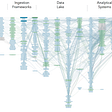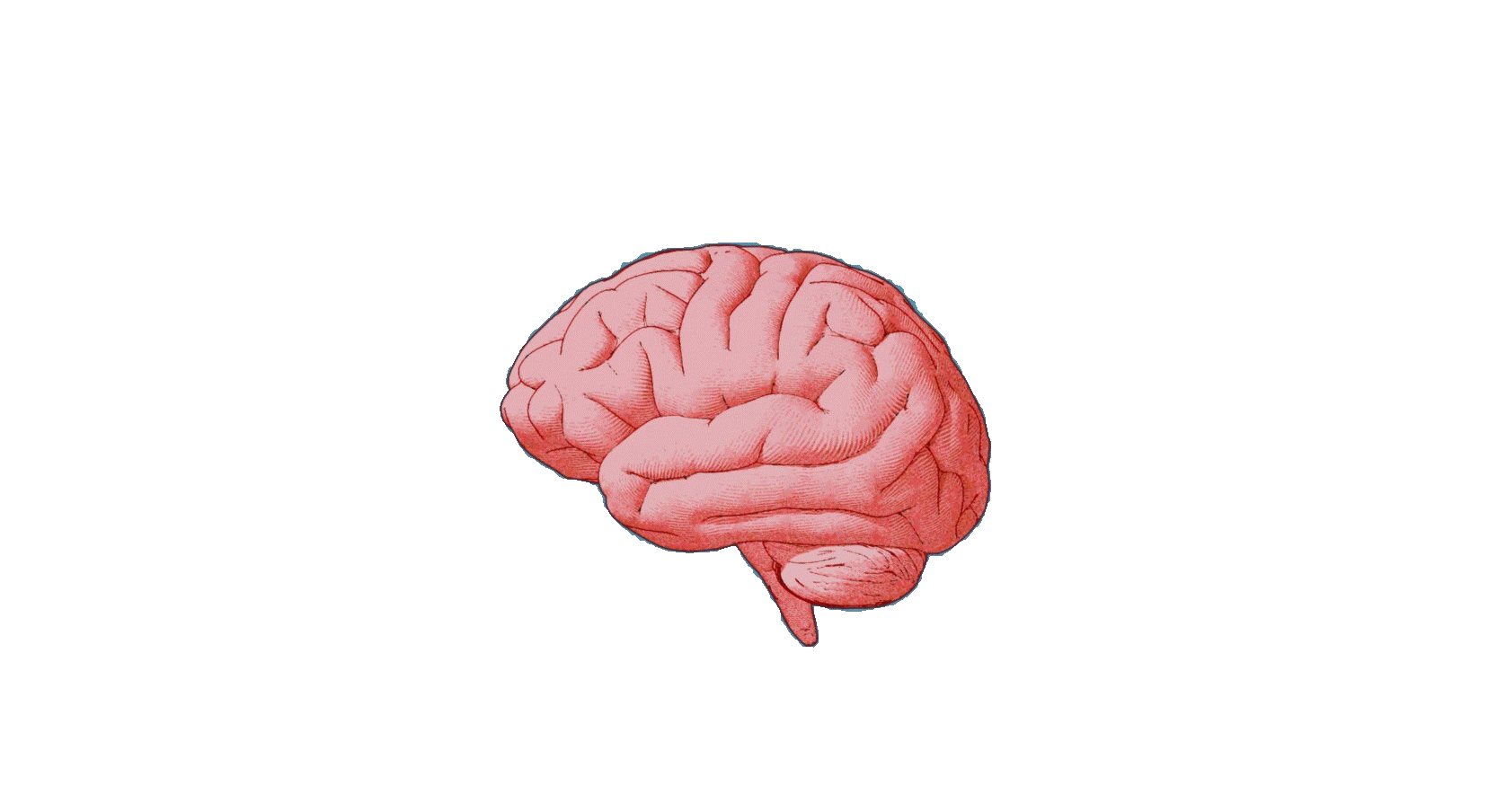# What is the Standard Normal Distribution and how do we interpret it?

What is so special about normal probability distribution? Why do so many data science and machine learning articles revolve around normal probability distribution?

The world of machine learning and data science revolves around the concepts of probability distributions and the core of the probability distribution concept is focused on Normal distributions. This article illustrates what normal distribution is and why it is widely used, in particular for a data scientist and a machine learning expert.

We have abundant data but data alone is not interesting. Data must be interpreted in order to add meaning. Data can be “distributed” (spread out) in different ways as follows:

There are cases where data is distributed like a “bell curve” where the data tends to be around a central value (i.e., mean) with no bias left or right, no multiple modes, and it gets close to a “Normal Distribution” (symmetrical and unimodal).

In above, the “Bell Curve” is a Normal Distribution and the blue histogram shows some data that follows it closely but not perfectly (which is usual). And the distribution is usually known as a bell curve because it looks like a bell.

## Examples that mainly follow a Normal Distribution

1. Blood pressure
2. Height of students in a class
3. Errors while taking measurements
4. Marks in a test, etc

## Some Basic Terminology

1. Mean(μ) — is the average of a data set.
2. Median — is the middle of the set of numbers.
3. Mode — is the most common number(peak) in a data set. A unimodal distribution only has one peak in the distribution, a bimodal distribution has two peaks, and a multimodal distribution has three or more peaks.
4. Bias — is the tendency of a statistic to overestimate or underestimate a parameter.

5. Skewness — refers to a distortion or asymmetry that deviates from the symmetrical bell curve, or normal distribution, in a set of data.

6. Standard deviation(σ) — is a measure of the amount of variation or dispersion of a set of values. A low standard deviation indicates that the values tend to be close to the mean of the set, while a high standard deviation indicates that the values are spread out over a wider range.

Example of IQ scores of students in a class:

## Characteristics of Normal Distribution

• mean = median = mode
• Unimodal
• 50% of values less than the mean and 50% greater than the mean

## The shape of the Normal Distribution

• 68.3% of values are within 1 standard deviation (1σ) of the mean
• 95.5% of values are within 2 standard deviations (2σ) of the mean
• 99.7% of values are within 3 standard deviations (3σ) of the mean

It is always good to know the standard deviation because we can say that any value is:

• likely to be within 1 standard deviation (1σ)(68.3 out of 100 should be)
• very likely to be within 2 standard deviations (2σ) (95.5 out of 100 should be)
• almost certainly within 3 standard deviations (3σ) (997 out of 1000 should be)

## 95.5% of students at school got marks in a test between 32 and 98.

Assuming this data is normally distributed we can calculate the mean and standard deviation

The mean is halfway between 32 and 98:

Mean(μ)= (32+98) / 2 = 65

95.5 % is 2 standard deviations on either side of the mean (a total of 4 standard deviations) so:

1 standard deviation (σ)= (98–32) /4 = 66 / 4 = 16.5

# Standard Normal Distribution

What is a “Z-score”?

The number of standard deviations from the mean is also called the “Standard Score”, “sigma” or “Z-score”. Simply, a Z-score describes the position of a raw score in terms of its distance from the mean, when measured in standard deviation units.

• Z is the “z-score” (Standard Score)
• x is the value to be standardized
• μ (mu) is the mean
• σ (sigma) is the standard deviation

A Z-score can be placed on a normal distribution curve. Z-scores range from -3 standard deviations (which would fall to the far left) up to +3 standard deviations (which would fall to the far right).

• Z-score = 0 — indicates that the data point’s score is identical to the mean score.
• Z-score=1.0 — indicate a value that is one standard deviation from the mean.
• Z-scores may be positive or negative, with a positive value indicating the score is above the mean and a negative score indicating it is below the mean.

## Standardizing

We can take any Normal Distribution and convert it to The Standard Normal Distribution.

The Standard Normal Distribution, also called the z-distribution, is a special normal distribution where the mean(μ) is 0 and the standard deviation(σ) is 1 and is denoted by Z(0,1).

## Why Standardize the values?

Let me explain this with the help of an example.

Suppose your teacher is marking your final exam of 100 marks with a passing criterion of 45 marks.

Below are the results of you and your classmates:

30, 75, 26, 72, 18, 44, 35, 12, 56, 33, 28

According to the above marks, most will fail and only 3 would pass !! 😰

The exam must have been really hard, so the teacher decides to Standardize all the scores and only fail people more than 1 standard deviation below the mean.😎

The Mean is 39, and the Standard Deviation is 19.4, and these are the Standard Scores:

-0.46, 1.85, -0.67, 1.70, -1.08, -0.26, -0.21,-1.39, 0.87, 0.21,-0.05

Now only 2 students will fail (< -1, the ones lower than −1 standard deviation since σ =1) 😇

Use the standard normal distribution to find the probability

The Standard Normal Distribution is a probability distribution, so the area under the curve between two points tells you the probability of variables taking on a range of values. The total area under the curve is 1 or 100%.

Every z-score has an associated p-value that tells you the probability of all values below or above that z-score occurring. This is the area under the curve left or right of that z-score.

This is how the Normal distribution is important in the world of data science and machine learning!!

If you liked the article, please hit the 👏 icon to support it. This will help other Medium users find it. Share it, so that others can read it!

Happy Learning! 😊

--

--

--

## More from Nerd For Tech

NFT is an Educational Media House. Our mission is to bring the invaluable knowledge and experiences of experts from all over the world to the novice. To know more about us, visit https://www.nerdfortech.org/.

## Data Lineage for Crisis## Algorithms For Data Scientists — Insertion Sort## A Brief Description of Data Lakes## 4 Blockers and 4 Unlockers for successful machine learning projects## Incident Management for Data Teams## Integrating Machine Learning Models within Matured Business Process## Observability @ Data Pipelines## Priyanka Dandale

Data Analyst at Infosys Ltd., AI Engineer, MSc. Statistics SPPU.

## More from Medium## What is Maximum Likelihood Estimation?## Point Estimates, Confidence Intervals, Z-Test vs T-Tests (One sample) [Part 1]## Random Brain